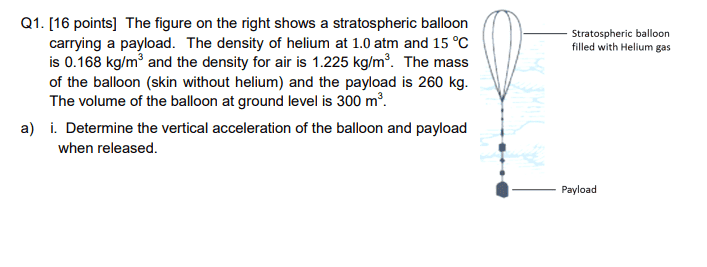# Stratospheric Balloon question

nicky670
Homework Statement:
The figure on the right shows a stratospheric balloon
carrying a payload. The density of helium at 1.0 atm and 15 oC
is 0.168 kg/m3 and the density for air is 1.225 kg/m3. The mass
of the balloon (skin without helium) and the payload is 260 kg.
The volume of the balloon at ground level is 300 m3
Relevant Equations:
Upthrust?
I have gathered the formula:
F(Bouyant force) - (Mballoon + Mpayload)g - (Mhelium)g = (Mballoon + Mhelium + Mpayload)a
But i can't seem to figure out what is the buoyant force to find a. Or am i mistaking something?Homework Helper
Gold Member
F(Bouyant force) - (Mballoon + Mpayload)g - (Mhelium)g = (Mballoon + Mhelium + Mpayload)a
Ok
But i can't seem to figure out what is the buoyant force to find a. Or am i mistaking something?
Have you covered Archimedes' Principle?

nicky670
Yes i have.

Homework Helper
Gold Member
According to Archimedes' principle, how would you find the buoyant force on a rock of volume V that is completely submerged in water?

•Chestermiller
nicky670
it is the volume of water displaced by the rock that will get you the buoyant force right?
According to Archimedes' principle, how would you find the buoyant force on a rock of volume V that is completely submerged in water?
It will be the volume of water displaced by the rock right? For buoyant force

Homework Helper
Gold Member
2022 Award
it is the volume of water displaced by the rock that will get you the buoyant force right?

It will be the volume of water displaced by the rock right? For buoyant force
A volume is not a force. What attribute of that volume of fluid?

nicky670
A volume is not a force. What attribute of that volume of fluid?
The weight of the volume of water displaced

Homework Helper
Gold Member
The weight of the volume of water displaced
Yes, that's right. Does this help with finding the buoyant force on the balloon?

nicky670
Yes, that's right. Does this help with finding the buoyant force on the balloon?
So i use rho = m/v formula to find the mass and then multiply by g=9.8 to get the weight?

Homework Helper
Gold Member
So i use rho = m/v formula to find the mass and then multiply by g=9.8 to get the weight?
That's the right idea. For what substance are you going to find the mass in order to get the buoyant force?